# NCERT Exemplar Solutions for Class 9 Maths Chapter 9 - Areas Of Parallelograms And Triangles

## NCERT Exemplar Solutions Class 9 Maths Chapter 9 – Free PDF Download

NCERT Exemplar Class 9 Maths Chapter 9 Areas of Parallelograms and Triangles, is provided here for students who are appearing for final exams. To encourage easy learning and help students understand the concepts of areas of parallelograms and triangles based on different scenarios, free NCERT exemplars are provided here which can be downloaded in the form of a PDF. Students can use these solved questions in exemplar solutions as a reference tool while practicing the NCERT book exercise questions, also. In this chapter, the topics covered are with respect to the CBSE Syllabus (2021-2022) prepared by our experts and they are as follows:

• Areas of parallelograms, on the same base and between the same parallels
• Areas of triangles on the same base and between the same Parallels
• Median of triangle dividing into two triangles

Learn to find the area of parallelogram and triangle by referring to NCERT Exemplar for Class 9 Maths Chapter 9 Areas of Parallelograms and Triangles. Solving the exemplar question will help students to learn important formulas and theorems of parallelograms and triangles the chapter in an easy and better way.

## Download PDF of NCERT Exemplar Solutions for Class 9 Maths Chapter 9 Areas of Parallelograms and Triangles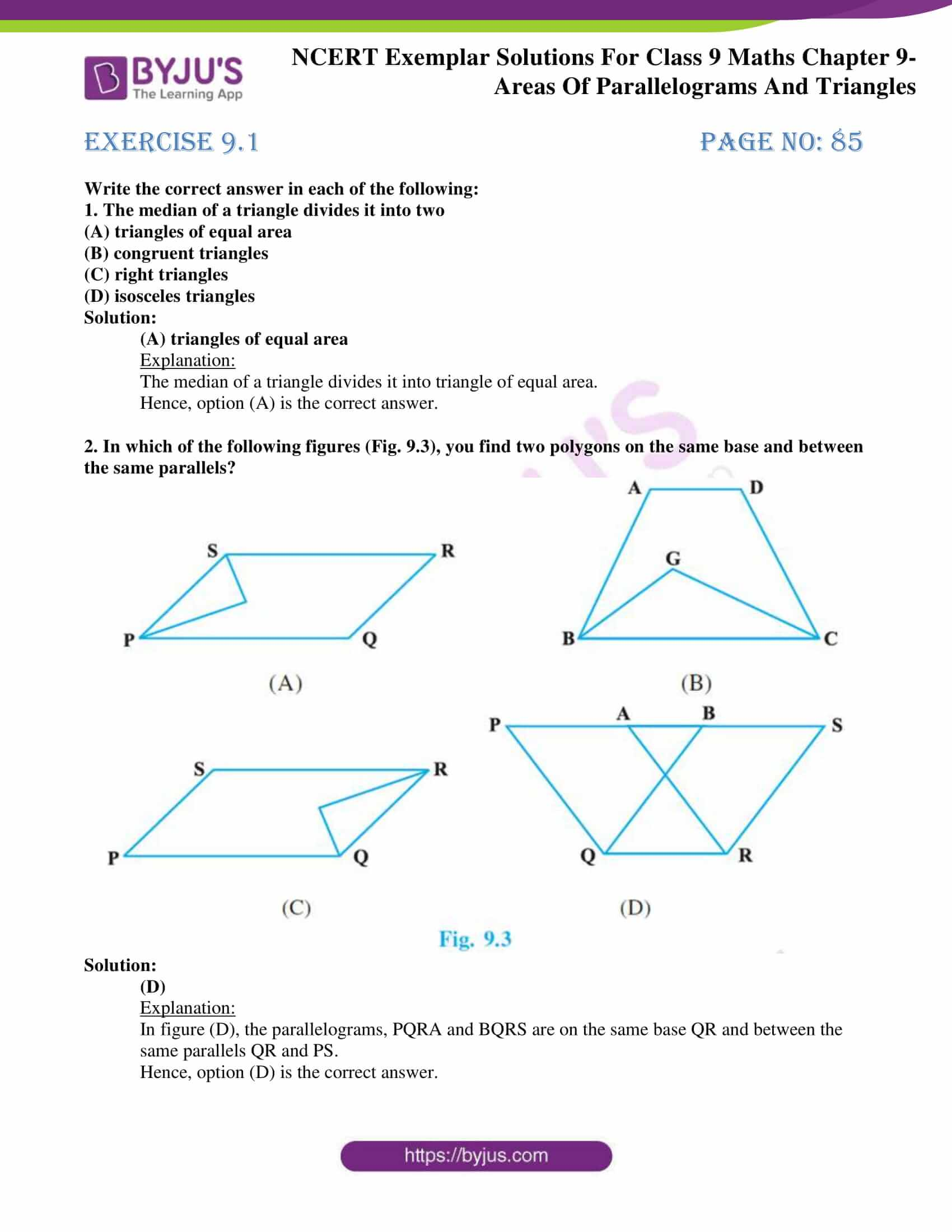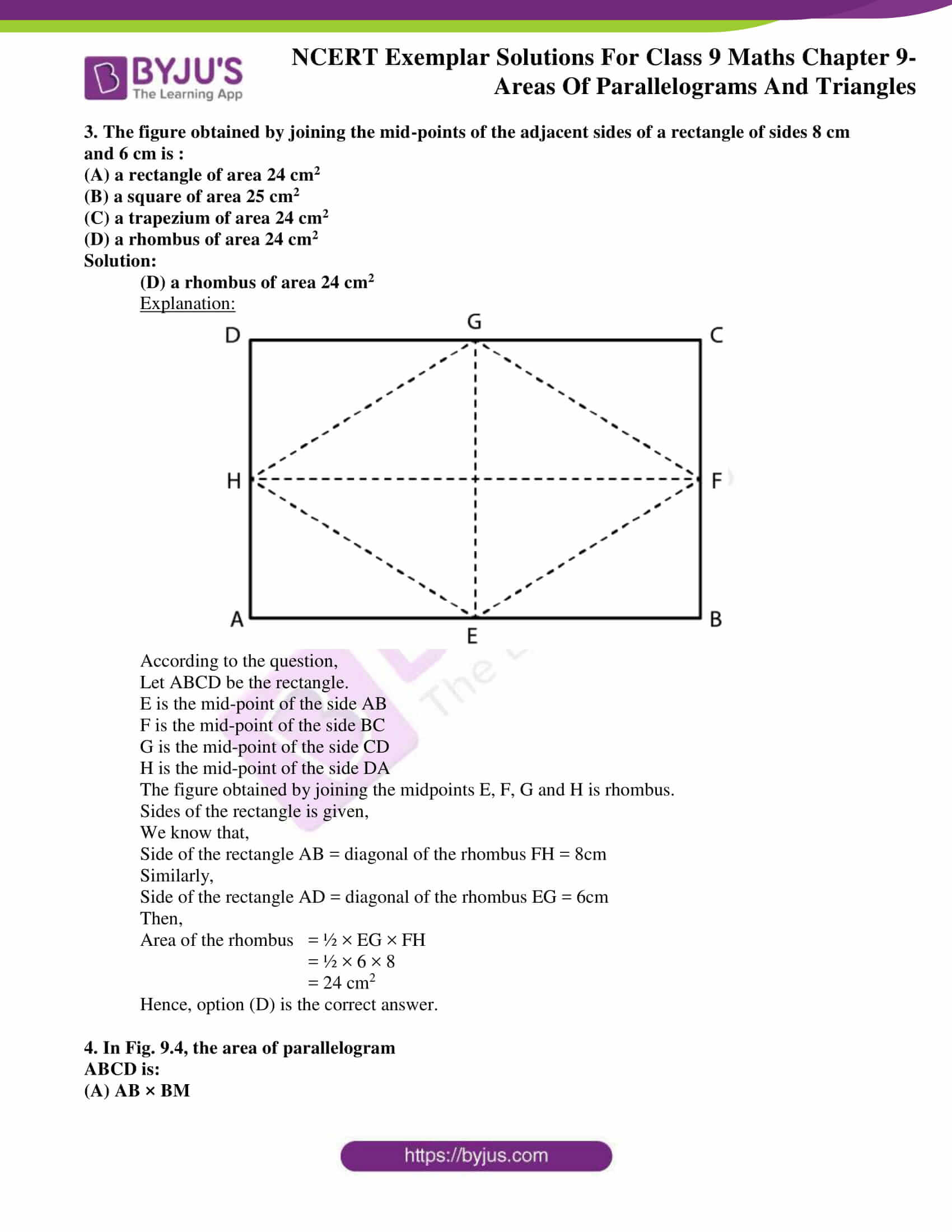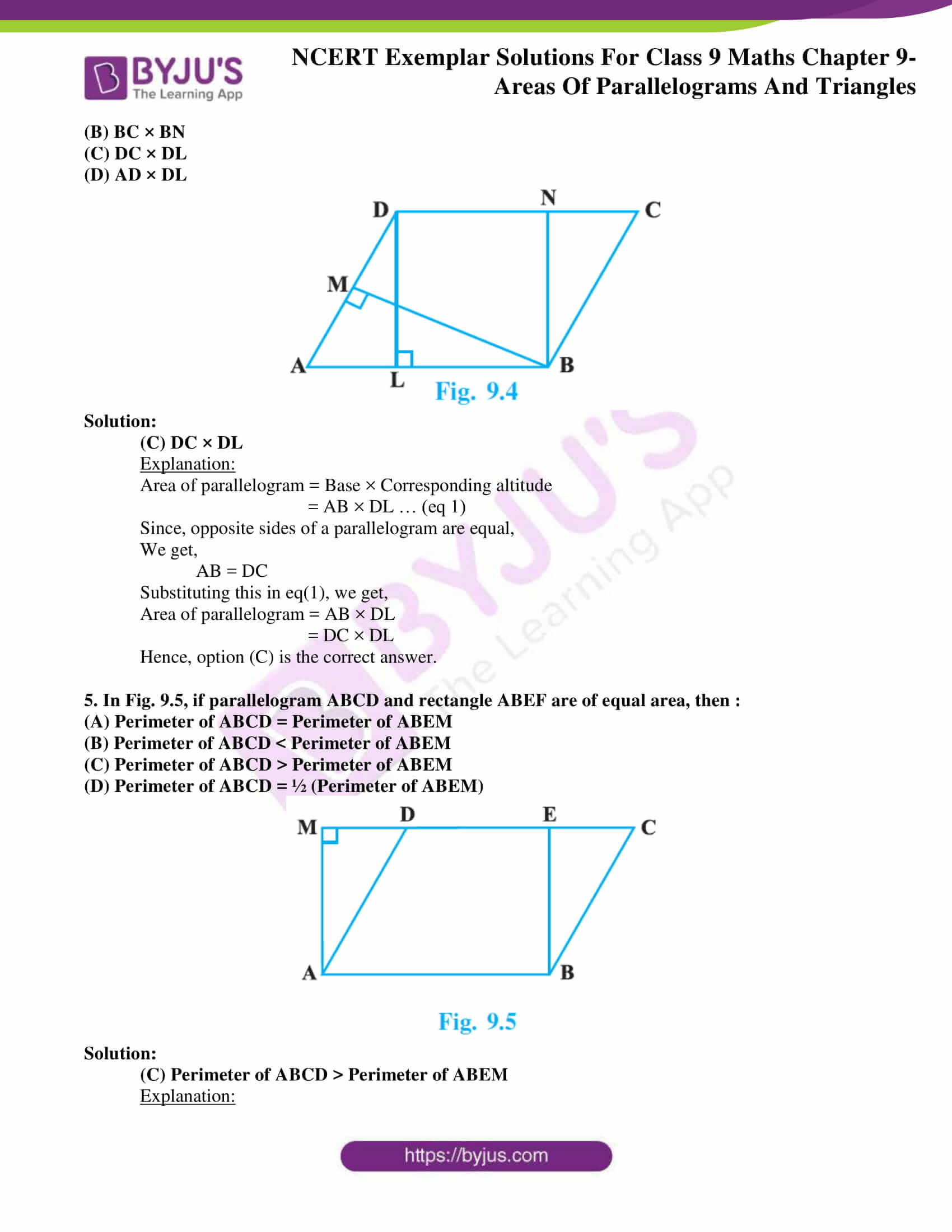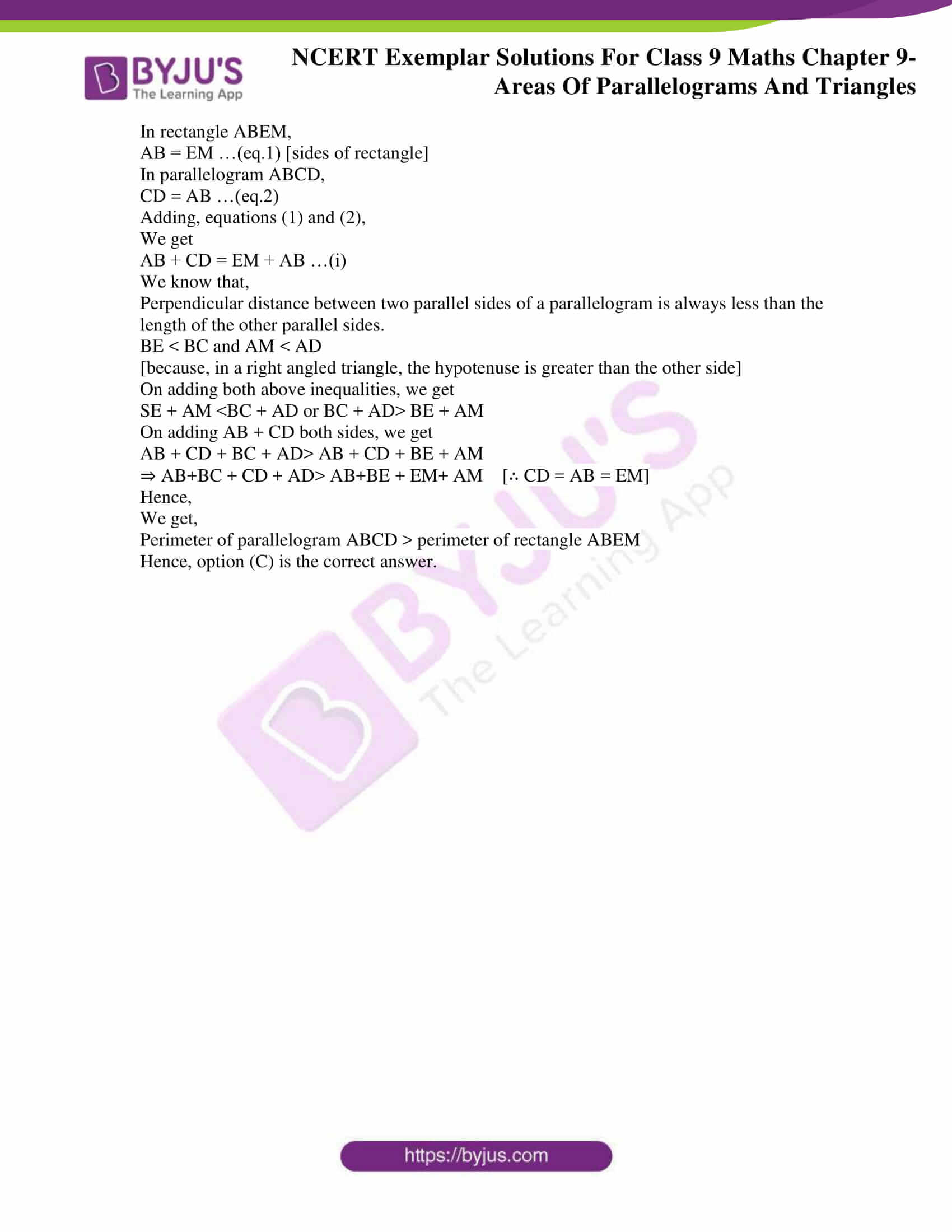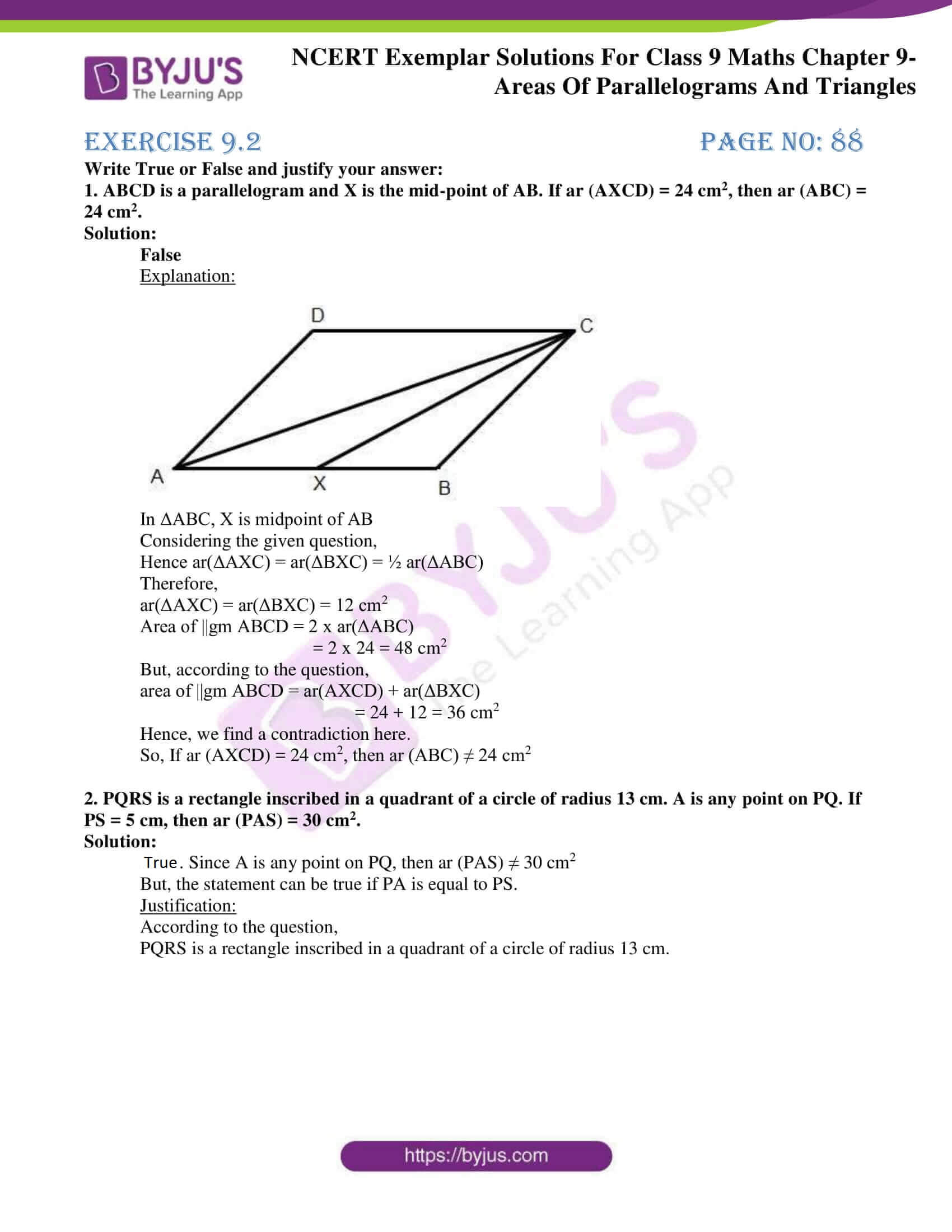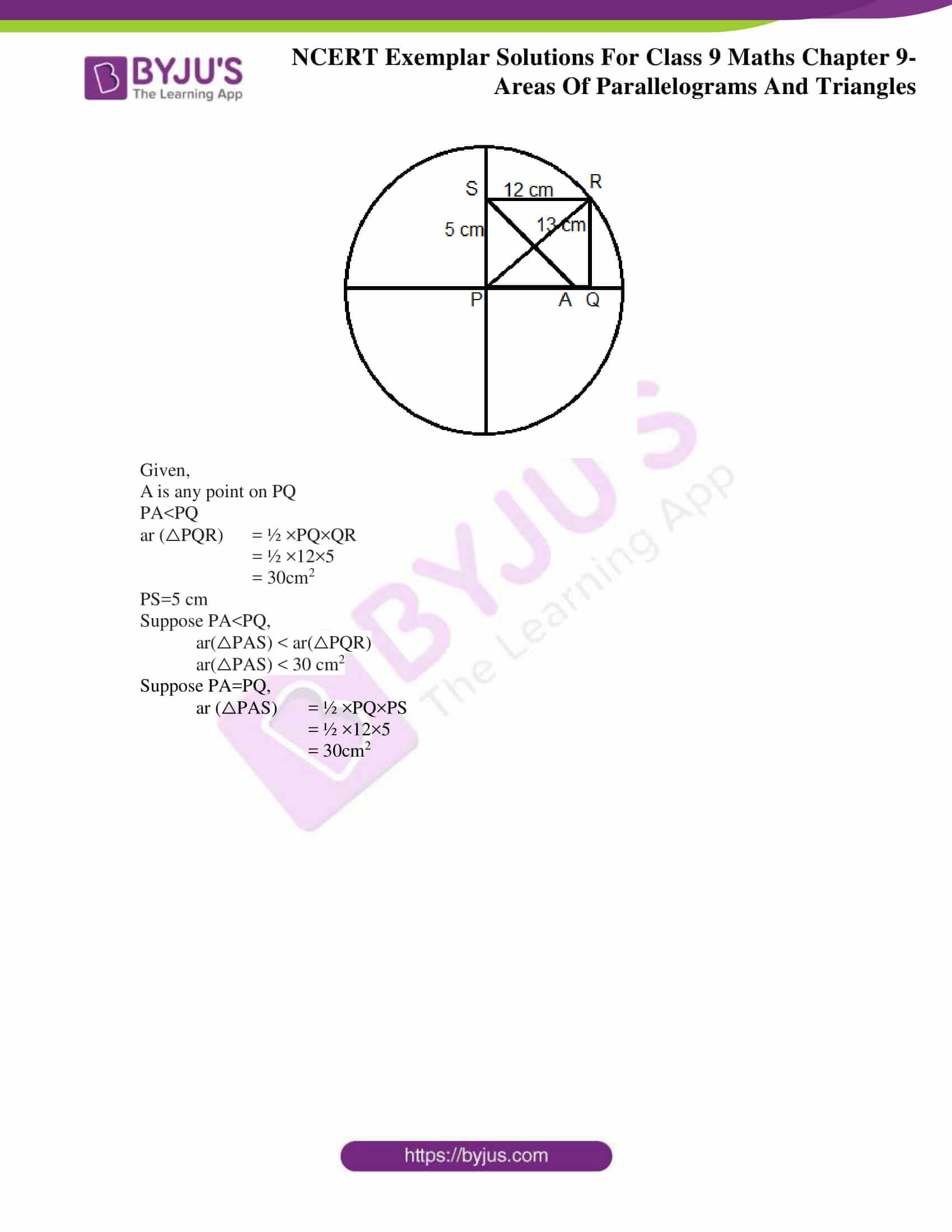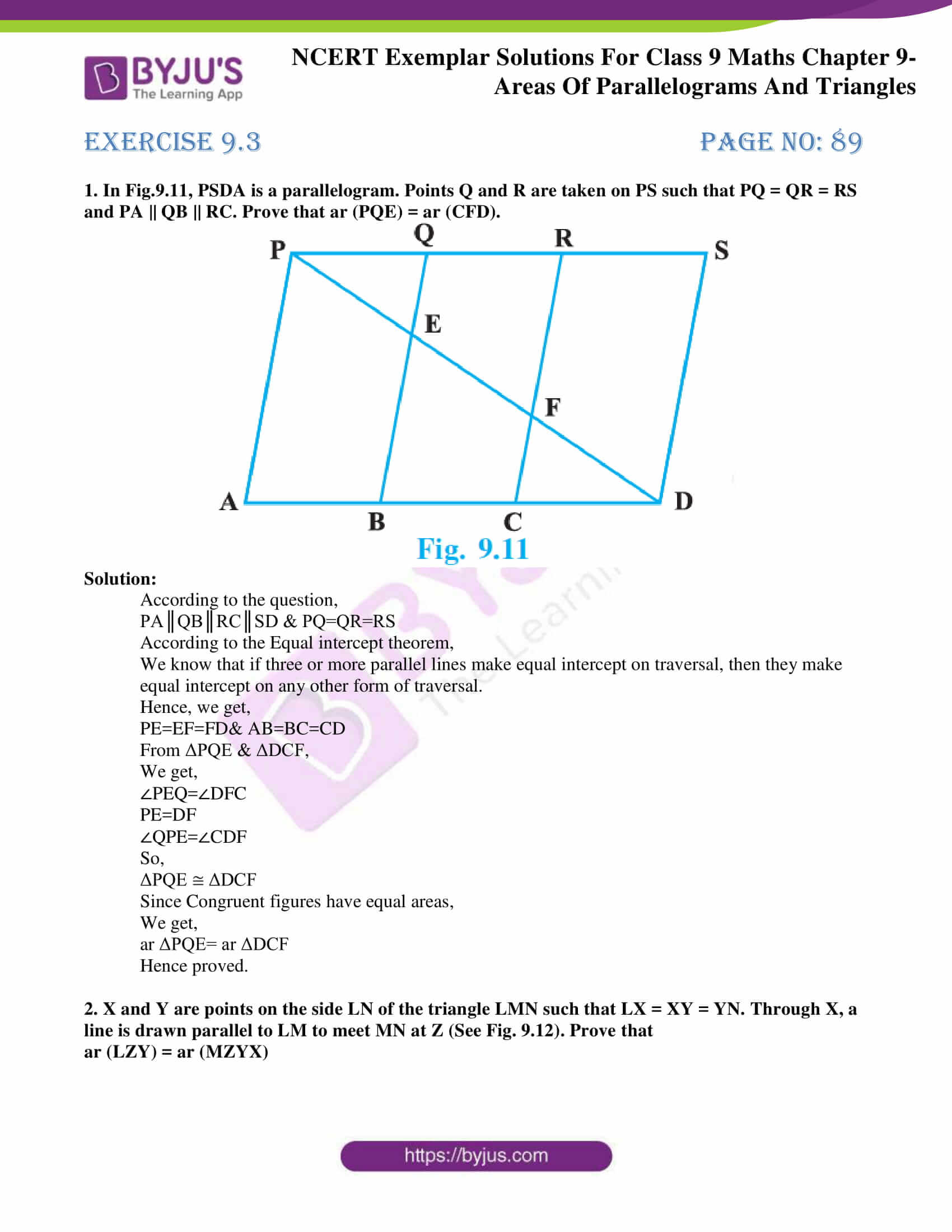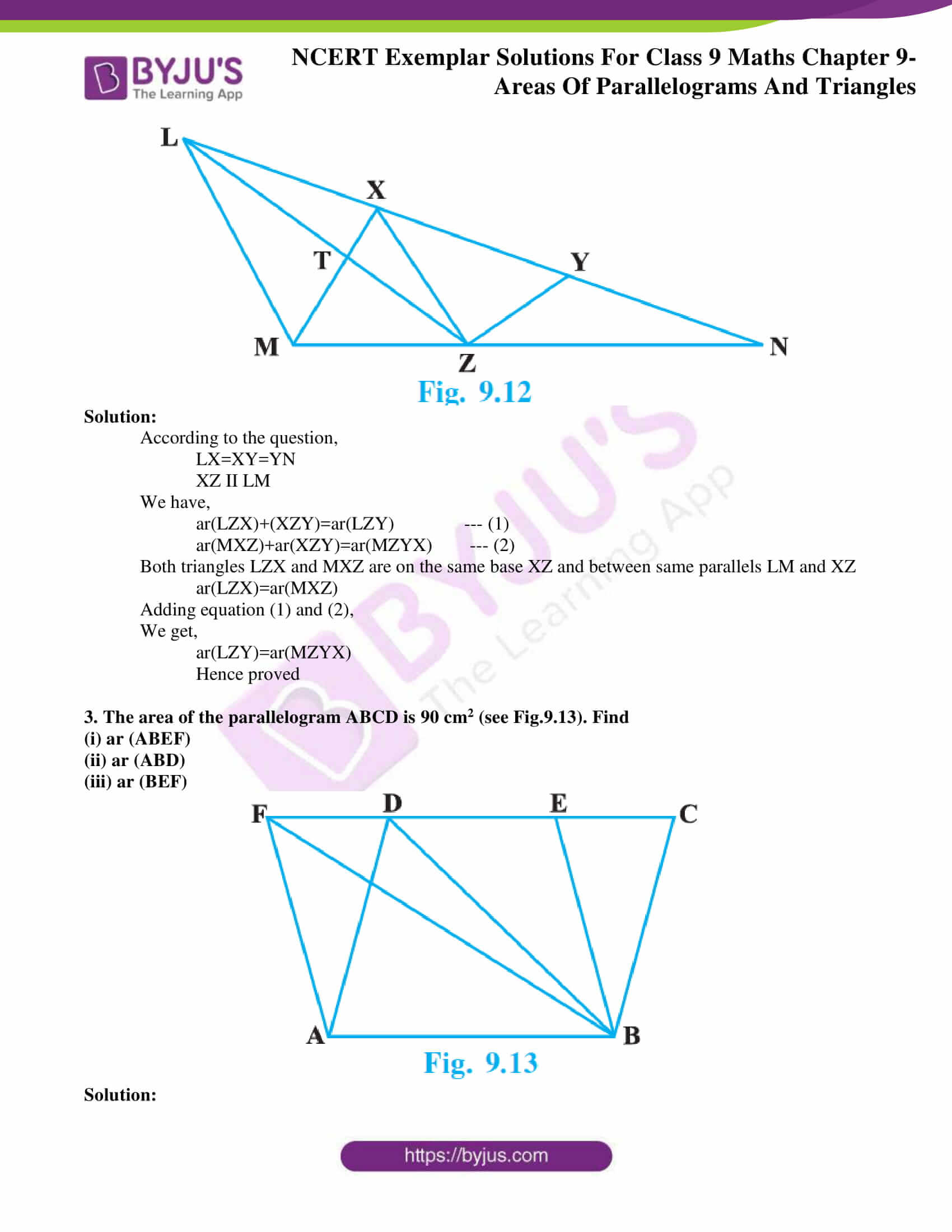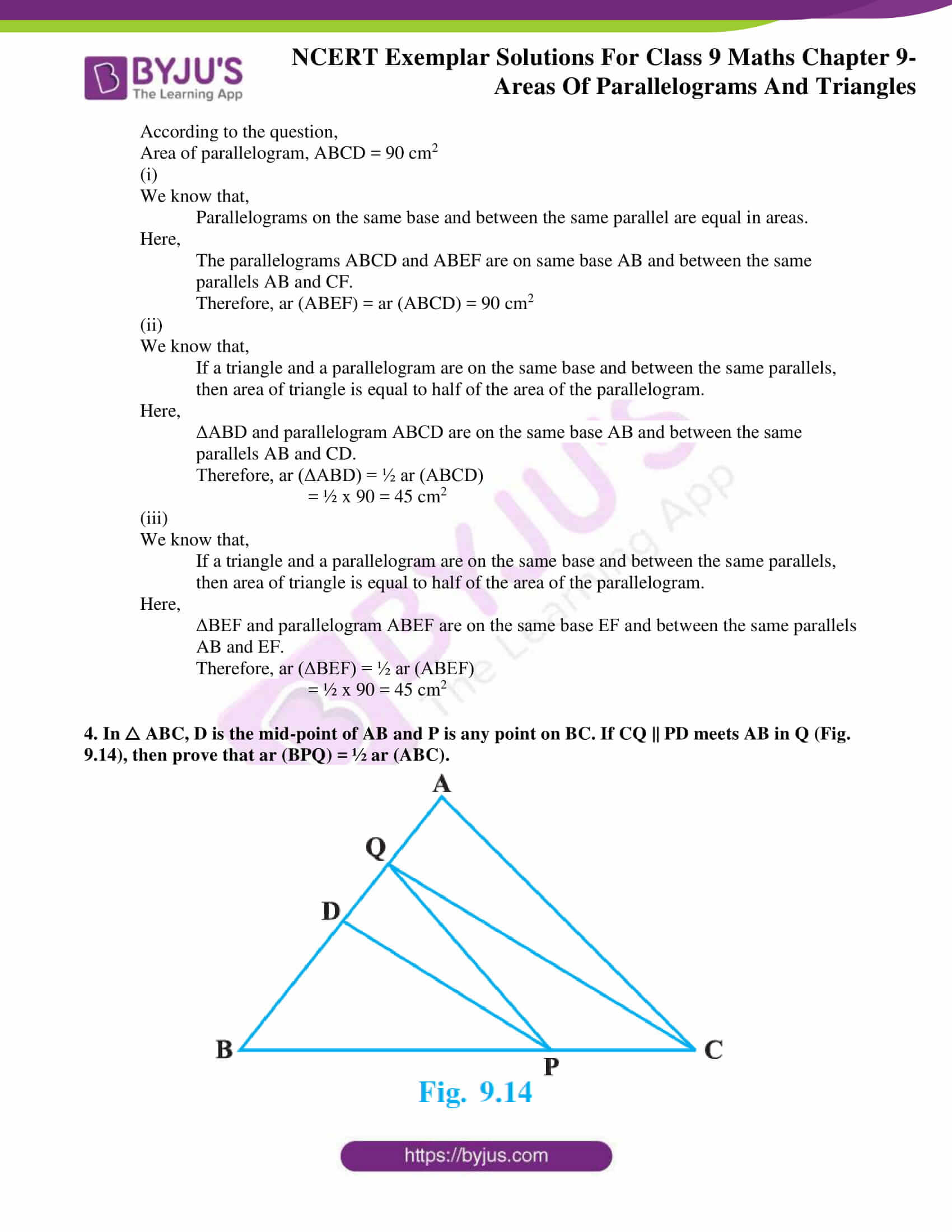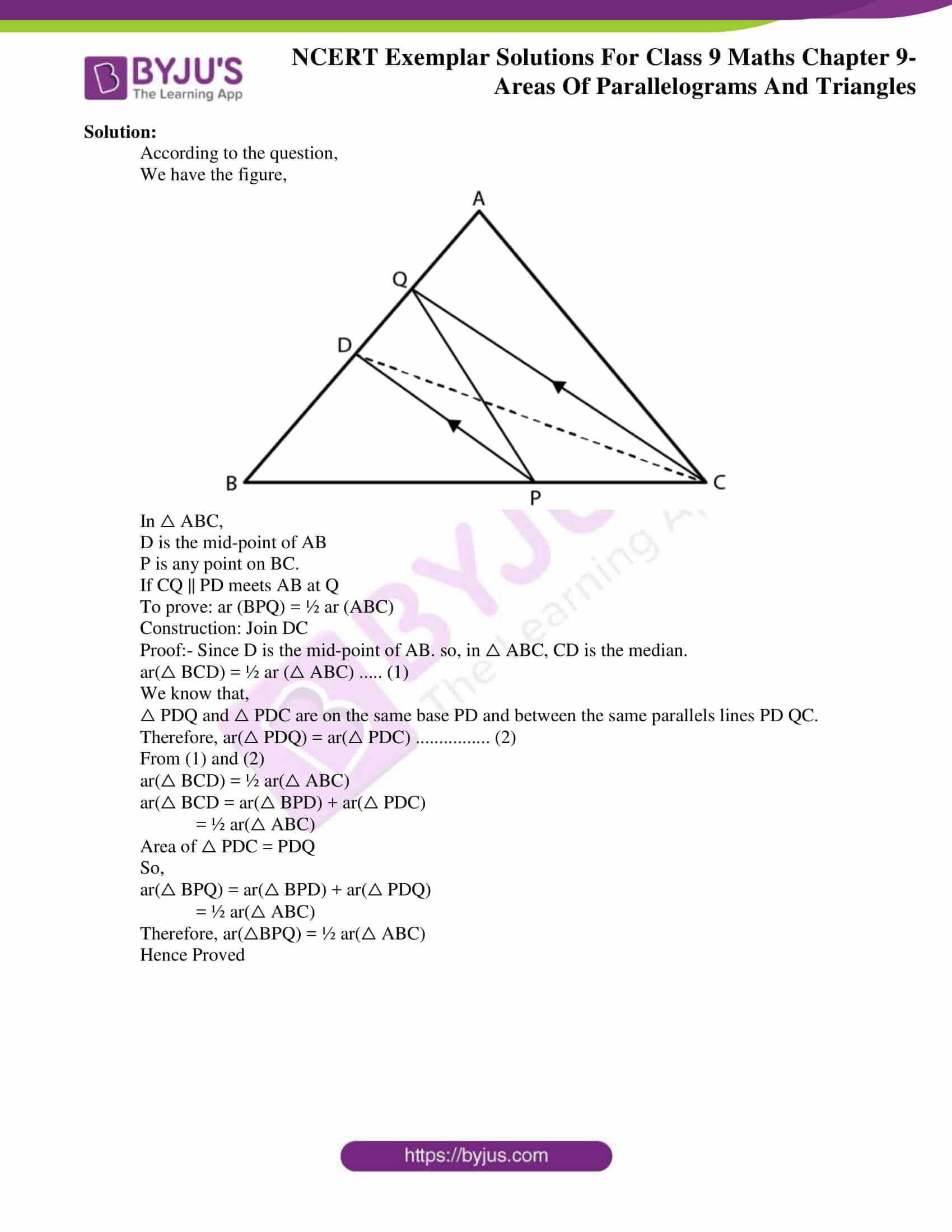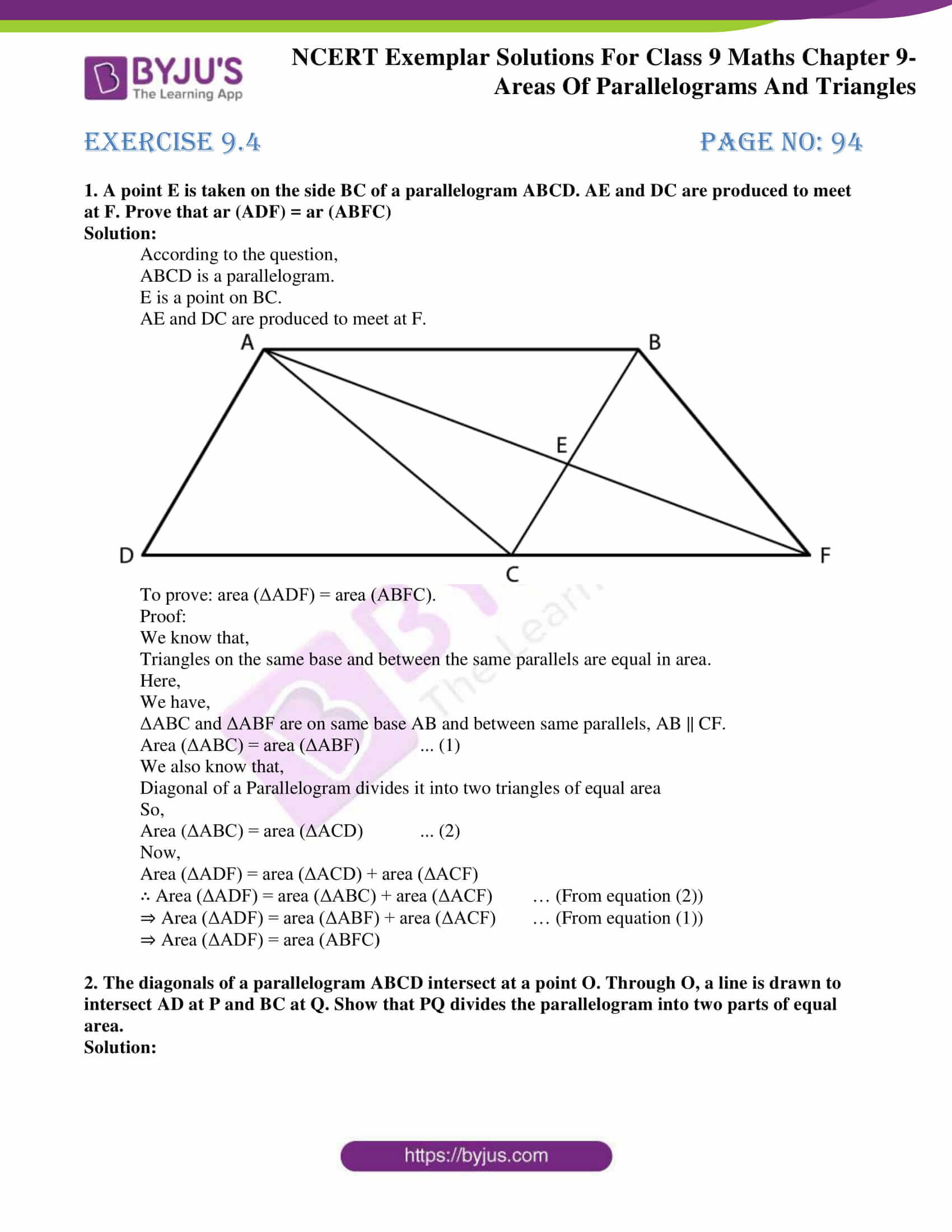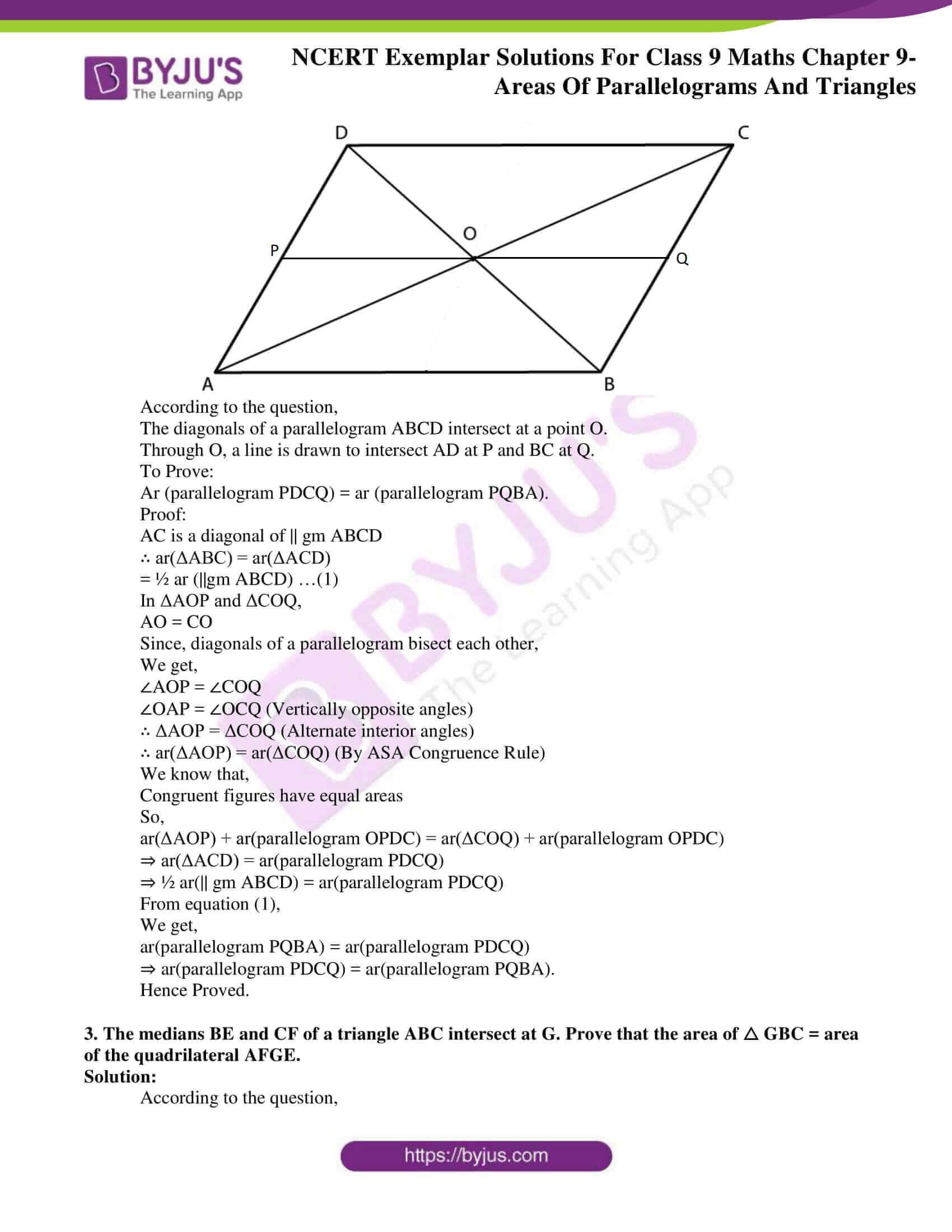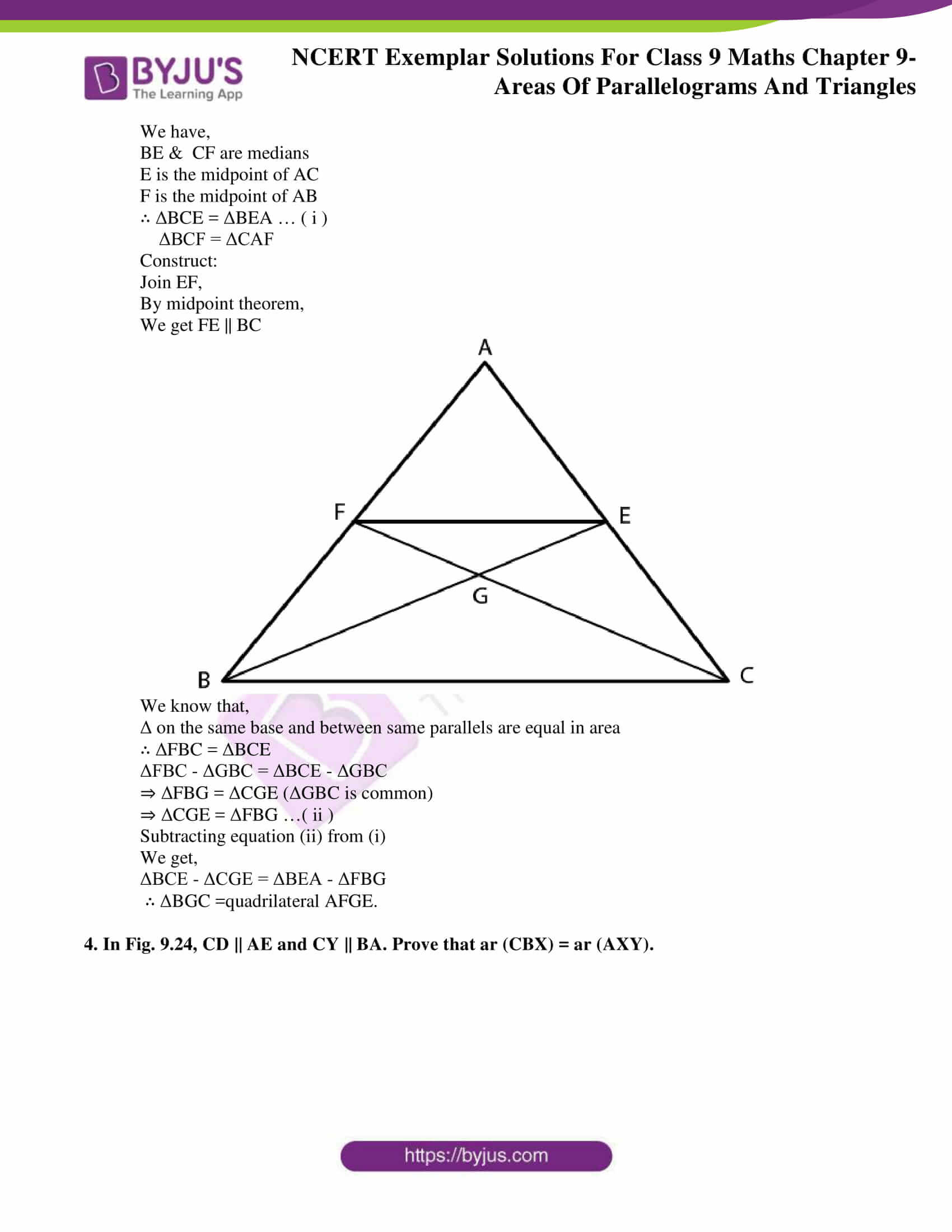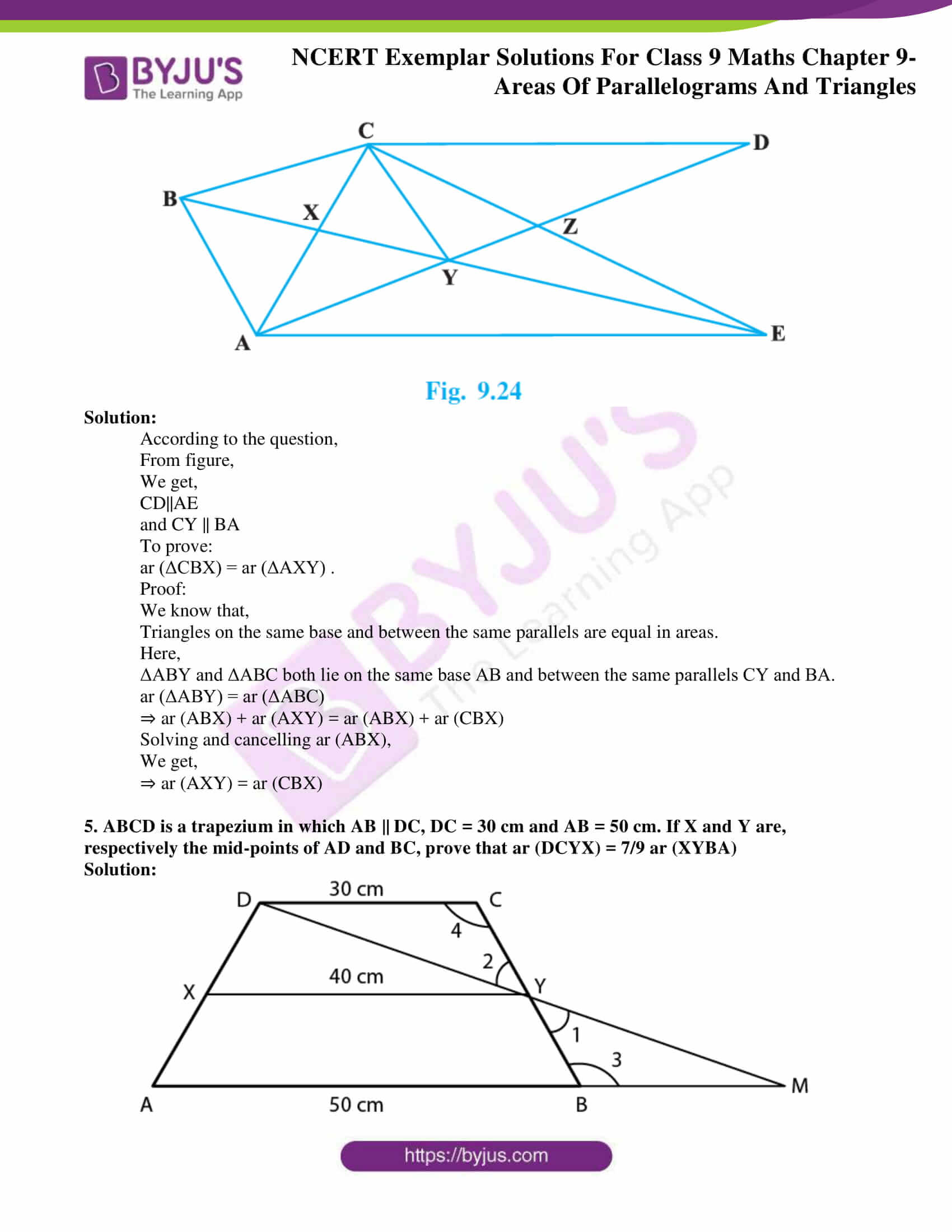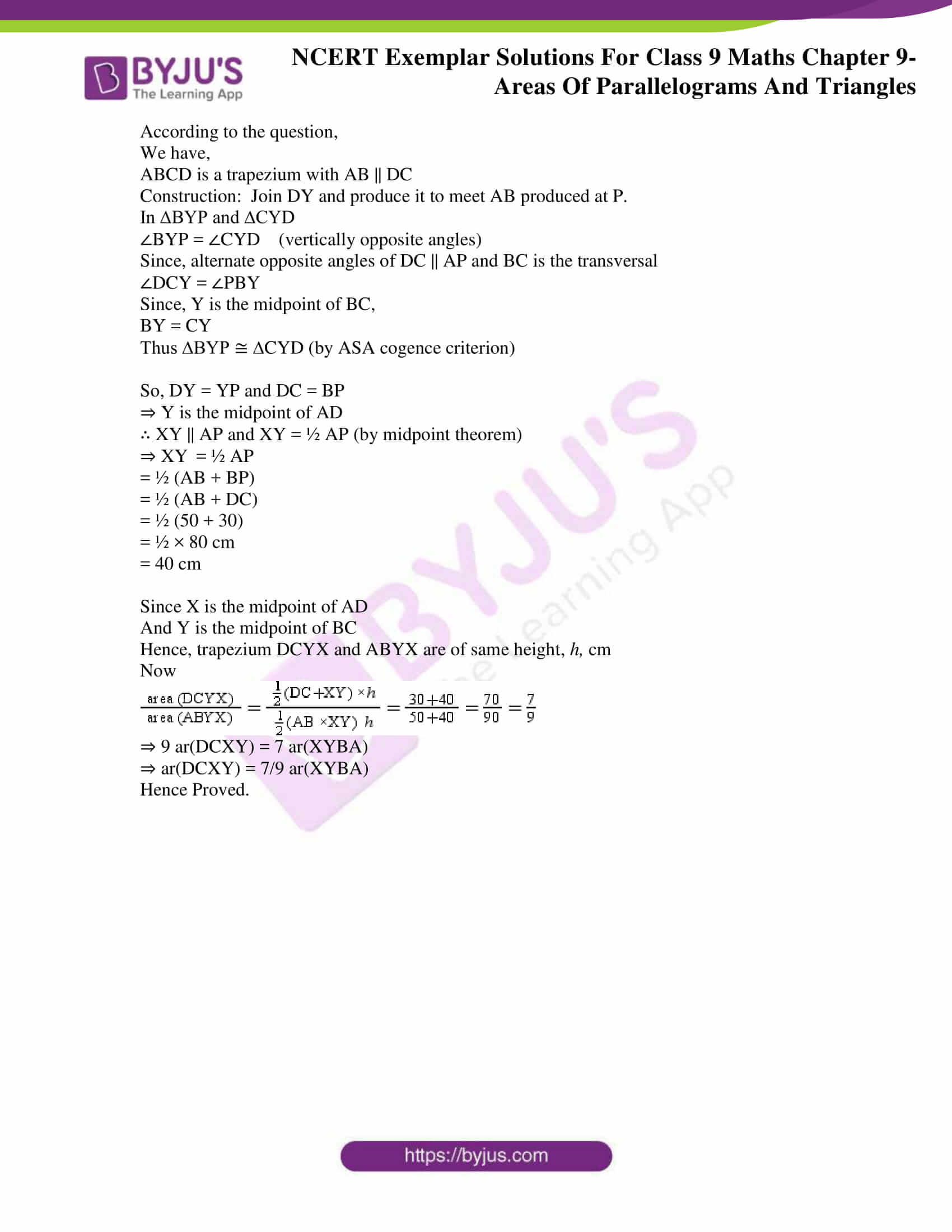### Access answers to NCERT Exemplar Solutions for Class 9 Maths Chapter 9 Areas of Parallelograms and Triangles

Exercise 9.1 Page No: 85

Write the correct answer in each of the following:

1. The median of a triangle divides it into two

(A) triangles of equal area

(B) congruent triangles

(C) right triangles

(D) isosceles triangles

Solution:

(A) triangles of equal area

Explanation:

The median of a triangle divides it into triangle of equal area.

Hence, option (A) is the correct answer.

2. In which of the following figures (Fig. 9.3), you find two polygons on the same base and between the same parallels?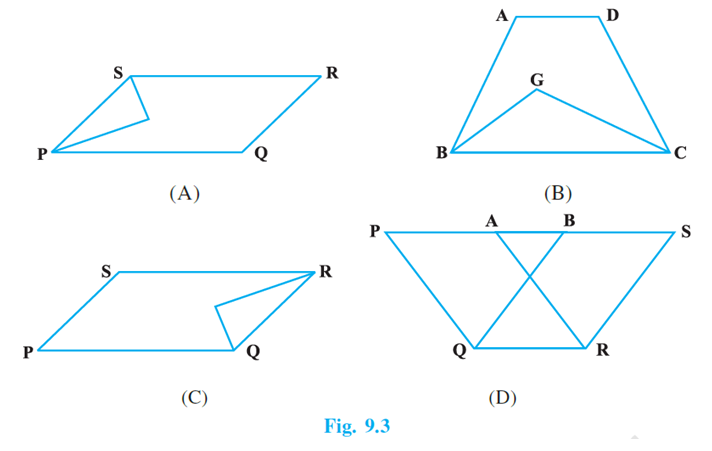Solution:

(D)

Explanation:

In figure (D), the parallelograms, PQRA and BQRS are on the same base QR and between the same parallels QR and PS.

Hence, option (D) is the correct answer.

3. The figure obtained by joining the mid-points of the adjacent sides of a rectangle of sides 8 cm and 6 cm is :

(A) a rectangle of area 24 cm2

(B) a square of area 25 cm2

(C) a trapezium of area 24 cm2

(D) a rhombus of area 24 cm2

Solution:

(D) a rhombus of area 24 cm2

Explanation: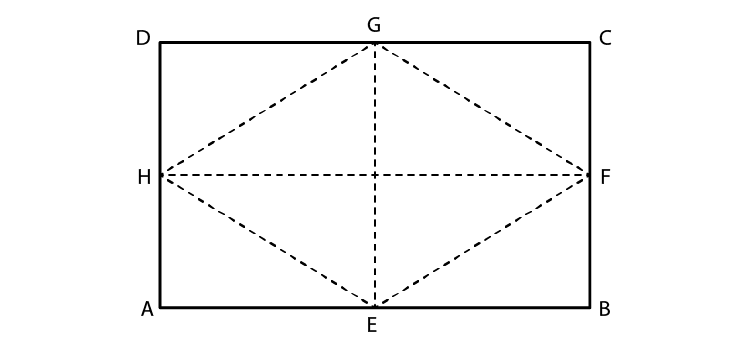According to the question,

Let ABCD be the rectangle.

E is the mid-point of the side AB

F is the mid-point of the side BC

G is the mid-point of the side CD

H is the mid-point of the side DA

The figure obtained by joining the midpoints E, F, G and H is rhombus.

Sides of the rectangle is given,

We know that,

Side of the rectangle AB = diagonal of the rhombus FH = 8cm

Similarly,

Side of the rectangle AD = diagonal of the rhombus EG = 6cm

Then,

Area of the rhombus = ½ × EG × FH

= ½ × 6 × 8

= 24 cm2

Hence, option (D) is the correct answer.

4. In Fig. 9.4, the area of parallelogram

ABCD is:

(A) AB × BM

(B) BC × BN

(C) DC × DL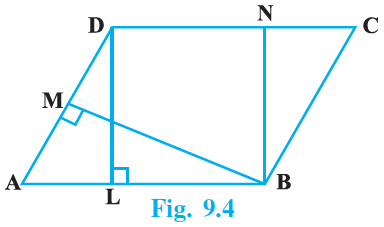Solution:

(C) DC × DL

Explanation:

Area of parallelogram = Base × Corresponding altitude

= AB × DL … (eq 1)

Since, opposite sides of a parallelogram are equal,

We get,

AB = DC

Substituting this in eq(1), we get,

Area of parallelogram = AB × DL

= DC × DL

Hence, option (C) is the correct answer.

5. In Fig. 9.5, if parallelogram ABCD and rectangle ABEF are of equal area, then :

(A) Perimeter of ABCD = Perimeter of ABEM

(B) Perimeter of ABCD < Perimeter of ABEM

(C) Perimeter of ABCD > Perimeter of ABEM

(D) Perimeter of ABCD = ½ (Perimeter of ABEM)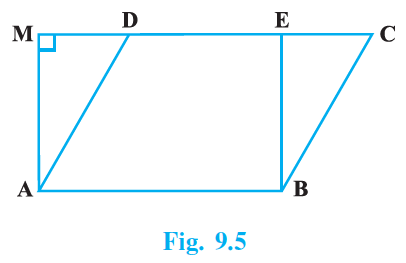Solution:

(C) Perimeter of ABCD > Perimeter of ABEM

Explanation:

In rectangle ABEM,

AB = EM …(eq.1) [sides of rectangle]

In parallelogram ABCD,

CD = AB …(eq.2)

We get

AB + CD = EM + AB …(i)

We know that,

Perpendicular distance between two parallel sides of a parallelogram is always less than the length of the other parallel sides.

BE < BC and AM < AD

[because, in a right angled triangle, the hypotenuse is greater than the other side]

On adding both above inequalities, we get

SE + AM <BC + AD or BC + AD> BE + AM

On adding AB + CD both sides, we get

AB + CD + BC + AD> AB + CD + BE + AM

⇒ AB+BC + CD + AD> AB+BE + EM+ AM    [∴ CD = AB = EM]

Hence,

We get,

Perimeter of parallelogram ABCD > perimeter of rectangle ABEM

Hence, option (C) is the correct answer.

Exercise 9.2 Page No: 88

1. ABCD is a parallelogram and X is the mid-point of AB. If ar (AXCD) = 24 cm2, then ar (ABC) = 24 cm2.

Solution:

False

Explanation: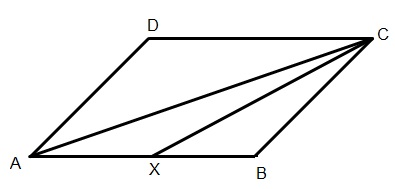In ΔABC, X is midpoint of AB

Considering the given question,

Hence ar(ΔAXC) = ar(ΔBXC) = ½ ar(ΔABC)

Therefore,

ar(ΔAXC) = ar(ΔBXC) = 12 cm2

Area of ||gm ABCD = 2 x ar(ΔABC)

= 2 x 24 = 48 cm2

But, according to the question,

area of ||gm ABCD = ar(AXCD) + ar(ΔBXC)

= 24 + 12 = 36 cm2

Hence, we find a contradiction here.

So, If ar (AXCD) = 24 cm2, then ar (ABC) ≠ 24 cm2

2. PQRS is a rectangle inscribed in a quadrant of a circle of radius 13 cm. A is any point on PQ. If PS = 5 cm, then ar (PAS) = 30 cm2.

Solution:

True. Since A is any point on PQ, then ar (PAS) ≠ 30 cm2

But, the statement can be true if PA is equal to PS.

Justification:

According to the question,

PQRS is a rectangle inscribed in a quadrant of a circle of radius 13 cm.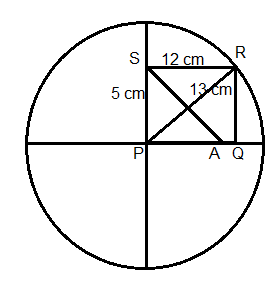Given,

A is any point on PQ

PA<PQ

ar (△PQR) = ½ ×PQ×QR

= ½ ×12×5

= 30cm2

PS=5 cm

Suppose PA<PQ,

ar(△PAS) < ar(△PQR)

ar(△PAS) < 30 cm2

Suppose PA=PQ,

ar (△PAS) = ½ ×PQ×PS

= ½ ×12×5

= 30cm2

Exercise 9.3 Page No: 89

1. In Fig.9.11, PSDA is a parallelogram. Points Q and R are taken on PS such that PQ = QR = RS and PA || QB || RC. Prove that ar (PQE) = ar (CFD).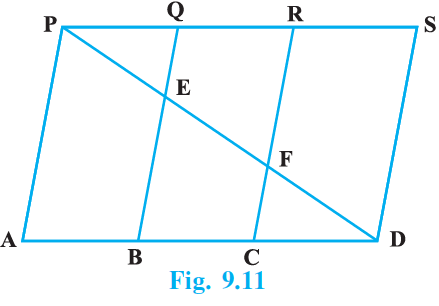Solution:

According to the question,

PA║QB║RC║SD & PQ=QR=RS

According to the Equal intercept theorem,

We know that if three or more parallel lines make equal intercept on traversal, then they make equal intercept on any other form of traversal.

Hence, we get,

PE=EF=FD& AB=BC=CD

From ΔPQE & ΔDCF,

We get,

∠PEQ=∠DFC

PE=DF

∠QPE=∠CDF

So,

ΔPQE ≅ ΔDCF

Since Congruent figures have equal areas,

We get,

ar ΔPQE= ar ΔDCF

Hence proved.

2. X and Y are points on the side LN of the triangle LMN such that LX = XY = YN. Through X, a line is drawn parallel to LM to meet MN at Z (See Fig. 9.12). Prove that

ar (LZY) = ar (MZYX)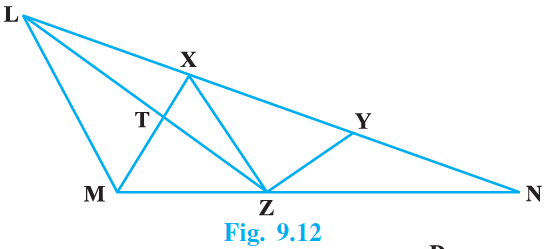Solution:

According to the question,

LX=XY=YN

XZ II LM

We have,

ar(LZX)+(XZY)=ar(LZY)               — (1)

ar(MXZ)+ar(XZY)=ar(MZYX)        — (2)

Both triangles LZX and MXZ are on the same base XZ and between same parallels LM and XZ

ar(LZX)=ar(MXZ)

We get,

ar(LZY)=ar(MZYX)

Hence proved

3. The area of the parallelogram ABCD is 90 cm2 (see Fig.9.13). Find

(i) ar (ABEF)

(ii) ar (ABD)

(iii) ar (BEF)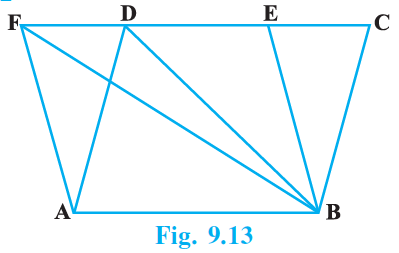Solution:

According to the question,

Area of parallelogram, ABCD = 90 cm2

(i)

We know that,

Parallelograms on the same base and between the same parallel are equal in areas.

Here,

The parallelograms ABCD and ABEF are on same base AB and between the same parallels AB and CF.

Therefore, ar (ABEF) = ar (ABCD) = 90 cm2

(ii)

We know that,

If a triangle and a parallelogram are on the same base and between the same parallels, then area of triangle is equal to half of the area of the parallelogram.

Here,

ΔABD and parallelogram ABCD are on the same base AB and between the same parallels AB and CD.

Therefore, ar (ΔABD) = ½ ar (ABCD)

= ½ x 90 = 45 cm2

(iii)

We know that,

If a triangle and a parallelogram are on the same base and between the same parallels, then area of triangle is equal to half of the area of the parallelogram.

Here,

ΔBEF and parallelogram ABEF are on the same base EF and between the same parallels AB and EF.

Therefore, ar (ΔBEF) = ½ ar (ABEF)

= ½ x 90 = 45 cm2

4. In △ ABC, D is the mid-point of AB and P is any point on BC. If CQ || PD meets AB in Q (Fig. 9.14), then prove that ar (BPQ) = ½ ar (ABC).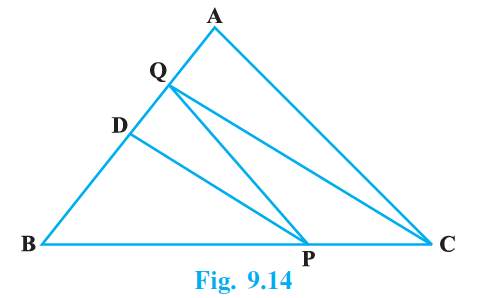Solution:

According to the question,

We have the figure,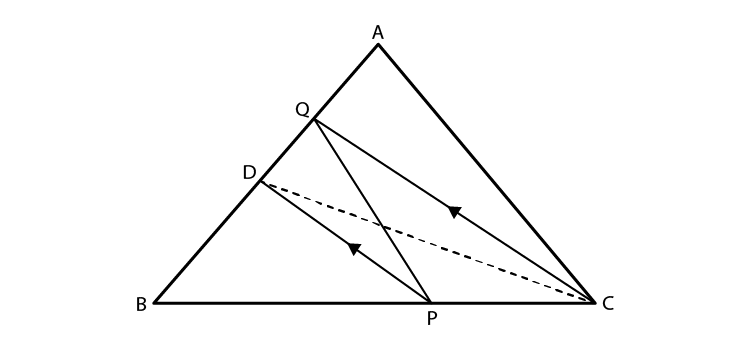In △ ABC,

D is the mid-point of AB

P is any point on BC.

If CQ || PD meets AB at Q

To prove: ar (BPQ) = ½ ar (ABC)

Construction: Join DC

Proof:- Since D is the mid-point of AB. so, in △ ABC, CD is the median.

ar(△ BCD) = ½ ar (△ ABC) ….. (1)

We know that,

△ PDQ and △ PDC are on the same base PD and between the same parallels lines PD QC.

Therefore, ar(△ PDQ) = ar(△ PDC) ……………. (2)

From (1) and (2)

ar(△ BCD) = ½ ar(△ ABC)

ar(△ BCD = ar(△ BPD) + ar(△ PDC)

= ½ ar(△ ABC)

Area of △ PDC = PDQ

So,

ar(△ BPQ) = ar(△ BPD) + ar(△ PDQ)

= ½ ar(△ ABC)

Therefore, ar(△BPQ) = ½ ar(△ ABC)

Hence Proved

Exercise 9.4 Page No: 94

1. A point E is taken on the side BC of a parallelogram ABCD. AE and DC are produced to meet at F. Prove that ar (ADF) = ar (ABFC)

Solution:

According to the question,

ABCD is a parallelogram.

E is a point on BC.

AE and DC are produced to meet at F.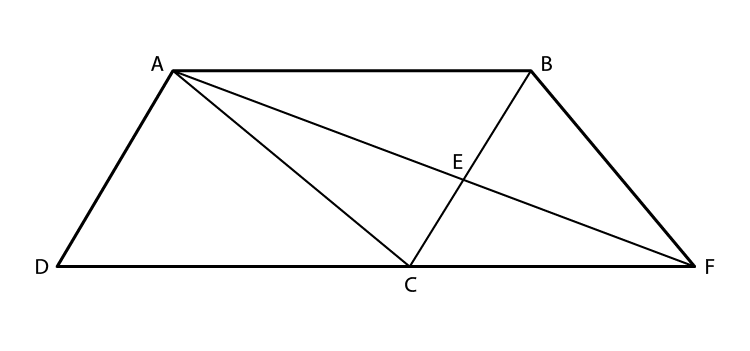To prove: area (ΔADF) = area (ABFC).

Proof:

We know that,

Triangles on the same base and between the same parallels are equal in area.

Here,

We have,

ΔABC and ΔABF are on same base AB and between same parallels, AB || CF.

Area (ΔABC) = area (ΔABF) … (1)

We also know that,

Diagonal of a Parallelogram divides it into two triangles of equal area

So,

Area (ΔABC) = area (ΔACD) … (2)

Now,

Area (ΔADF) = area (ΔACD) + area (ΔACF)

∴ Area (ΔADF) = area (ΔABC) + area (ΔACF) … (From equation (2))

⇒ Area (ΔADF) = area (ΔABF) + area (ΔACF) … (From equation (1))

⇒ Area (ΔADF) = area (ABFC)

2. The diagonals of a parallelogram ABCD intersect at a point O. Through O, a line is drawn to intersect AD at P and BC at Q. Show that PQ divides the parallelogram into two parts of equal area.

Solution: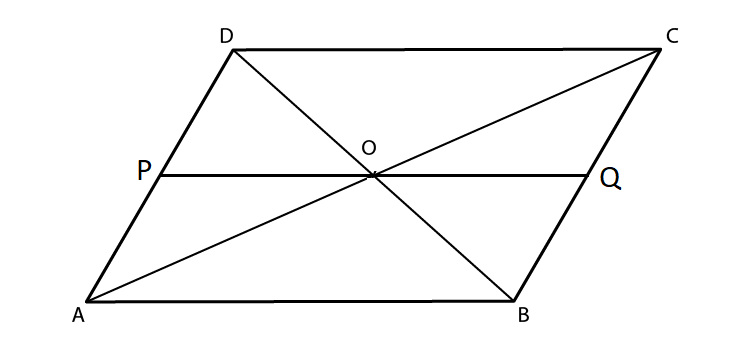According to the question,

The diagonals of a parallelogram ABCD intersect at a point O.

Through O, a line is drawn to intersect AD at P and BC at Q.

To Prove:

Ar (parallelogram PDCQ) = ar (parallelogram PQBA).

Proof:

AC is a diagonal of || gm ABCD

∴ ar(ΔABC) = ar(ΔACD)

= ½ ar (||gm ABCD) …(1)

In ΔAOP and ΔCOQ,

AO = CO

Since, diagonals of a parallelogram bisect each other,

We get,

∠AOP = ∠COQ

∠OAP = ∠OCQ (Vertically opposite angles)

∴ ΔAOP = ΔCOQ (Alternate interior angles)

∴ ar(ΔAOP) = ar(ΔCOQ) (By ASA Congruence Rule)

We know that,

Congruent figures have equal areas

So,

ar(ΔAOP) + ar(parallelogram OPDC) = ar(ΔCOQ) + ar(parallelogram OPDC)

⇒ ar(ΔACD) = ar(parallelogram PDCQ)

⇒ ½ ar(|| gm ABCD) = ar(parallelogram PDCQ)

From equation (1),

We get,

ar(parallelogram PQBA) = ar(parallelogram PDCQ)

⇒ ar(parallelogram PDCQ) = ar(parallelogram PQBA).

Hence Proved.

3. The medians BE and CF of a triangle ABC intersect at G. Prove that the area of △ GBC = area of the quadrilateral AFGE.

Solution:

According to the question,

We have,

BE &  CF are medians

E is the midpoint of AC

F is the midpoint of AB

∴ ΔBCE = ΔBEA … ( i )

ΔBCF = ΔCAF

Construct:

Join EF,

By midpoint theorem,

We get FE || BC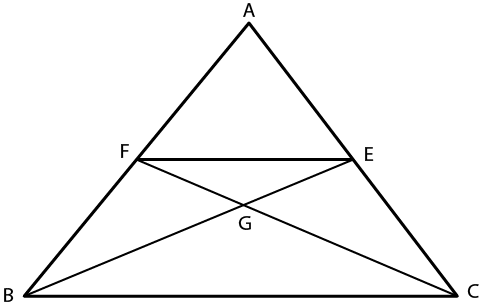We know that,

Δ on the same base and between same parallels are equal in area

∴ ΔFBC = ΔBCE

ΔFBC – ΔGBC = ΔBCE – ΔGBC

⇒ ΔFBG = ΔCGE (ΔGBC is common)

⇒ ΔCGE = ΔFBG …( ii )

Subtracting equation (ii) from (i)

We get,

ΔBCE – ΔCGE = ΔBEA – ΔFBG

4. In Fig. 9.24, CD || AE and CY || BA. Prove that ar (CBX) = ar (AXY).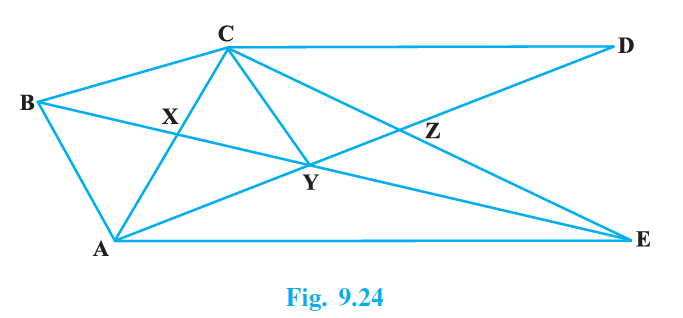Solution:

According to the question,

From figure,

We get,

CD||AE

and CY || BA

To prove:

ar (ΔCBX) = ar (ΔAXY) .

Proof:

We know that,

Triangles on the same base and between the same parallels are equal in areas.

Here,

ΔABY and ΔABC both lie on the same base AB and between the same parallels CY and BA.

ar (ΔABY) = ar (ΔABC)

⇒ ar (ABX) + ar (AXY) = ar (ABX) + ar (CBX)

Solving and cancelling ar (ABX),

We get,

⇒ ar (AXY) = ar (CBX)

5. ABCD is a trapezium in which AB || DC, DC = 30 cm and AB = 50 cm. If X and Y are, respectively the mid-points of AD and BC, prove that ar (DCYX) = 7/9 ar (XYBA)

Solution: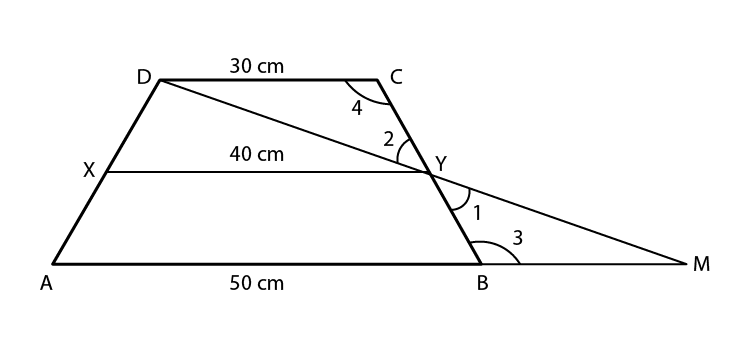According to the question,

We have,

ABCD is a trapezium with AB || DC

Construction:  Join DY and produce it to meet AB produced at P.

In ∆BYP and ∆CYD

∠BYP = ∠CYD    (vertically opposite angles)

Since, alternate opposite angles of DC || AP and BC is the transversal

∠DCY = ∠PBY

Since, Y is the midpoint of BC,

BY = CY

Thus ∆BYP ≅ ∆CYD (by ASA cogence criterion)

So, DY = YP and DC = BP

⇒ Y is the midpoint of AD

∴ XY || AP and XY = ½ AP (by midpoint theorem)

⇒ XY = ½ AP

= ½ (AB + BP)

= ½ (AB + DC)

= ½ (50 + 30)

= ½ × 80 cm

= 40 cm

Since X is the midpoint of AD

And Y is the midpoint of BC

Hence, trapezium DCYX and ABYX are of same height, h, cm

Now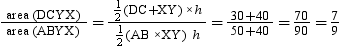⇒ 9 ar(DCXY) = 7 ar(XYBA)

⇒ ar(DCXY) = 7/9 ar(XYBA)

Hence Proved.

BYJU’S provide exemplar books, NCERT Solutions, notes, and question papers as learning materials for students so that they can practice a variety of Maths problems here resolving their difficulties. Students are also advised to solve sample papers and previous year question papers to know the type of questions asked from Maths Chapter 9, areas of parallelograms and triangles, and marks contained by it.

Keep reading with us and get exemplars for all the chapters of Maths Class 9. Download the BYJU’S app to get personalized video content and learn the concepts of maths such as Areas of Parallelograms and Triangles, in an easy way.

## Frequently Asked Questions on NCERT Exemplar Solutions for Class 9 Maths Chapter 9

### Explain the concept of parallelogram discussed in the Chapter 9 of NCERT Exemplar Solutions for Class 9 Maths?

A parallelogram is a two-dimensional geometrical shape, whose sides are parallel to each other. It is a type of polygon having four sides (also called quadrilateral), where the pair of parallel sides are equal in length. Also, the interior opposite angles of a parallelogram are equal in measure. Sum of adjacent angles of a parallelogram is equal to 180 degrees. To understand these concepts in a better way, students should refer to the NCERT Solutions designed by the expert faculty at BYJU’S. Even though the textbook has enough details, opting for the best study material will help students in understanding their applications.

### List out the concepts present in Chapter 9 of NCERT Exemplar Solutions for Class 9 Maths?

The concepts present in the Chapter 9 of NCERT Exemplar Solutions for Class 9 Maths are –
1. Areas of parallelograms, on the same base and between the same parallels
2. Areas of triangles on the same base and between the same Parallels
3. Median of triangle dividing into two triangles

### How to secure full marks in the Chapter 9 of the NCERT Exemplar Solutions for Class 9 Maths?

Chapter 9 requires a lot of practice to reduce the conceptual errors as it contains lots of difficult topics. The fundamental concepts might be a little tricky in the starting but with the right guidance securing a good score is not that difficult. If your aim is to score good marks in this chapter, you should solve different types of tricky problems as well. The NCERT Exemplar Solutions has fast solving tips which keeps students aware of the type of problems that would appear in the annual exams.